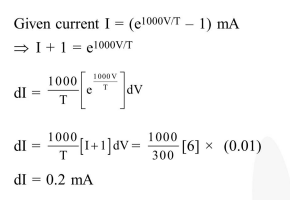Deepak Scored 45->99%ile with Bounce Back Crack Course. You can do it too!

# The current voltage relation of diode is given by

Question:

The current voltage relation of diode is given by $\mathrm{I}=\left(\mathrm{e}^{1000 \mathrm{~V} / \mathrm{T}}-1\right) \mathrm{mA}$, where the applied voltage $\mathrm{V}$ is in volts and the temperature $\mathrm{T}$ is in degree Kelvin. If a student makes an error measuring $\pm 0.01 \mathrm{~V}$ while measuring the current of $5 \mathrm{~mA}$ at $300 \mathrm{~K}$, what will be error in the value of current in mA?

1. $0.5 \mathrm{~mA}$

2. $0.05 \mathrm{~mA}$

3. $0.2 \mathrm{~mA}$

4. $0.02 \mathrm{~mA}$

Correct Option: , 3

Solution: